# Conductor rolling down an inclined plane in a vertical B-field

• ChiralSuperfields
In summary, the problem involves finding the value of theta in terms of phi using the magnetic force cross product formula. However, the given equation for this is incorrect and the direction of the magnetic force needs to be considered. It is unclear if the roller is sliding or rolling along the rails and this may affect the calculation. The problem statement also mentions a battery, but it is not clear if it is part of the circuit. There is a possibility of replacing the battery with a resistor R, which is included in the target expression. The case of constant velocity may also affect the solution, depending on if the roller is sliding or rolling.

#### ChiralSuperfields

Homework Statement
Relevant Equations
For part (c) of this problem,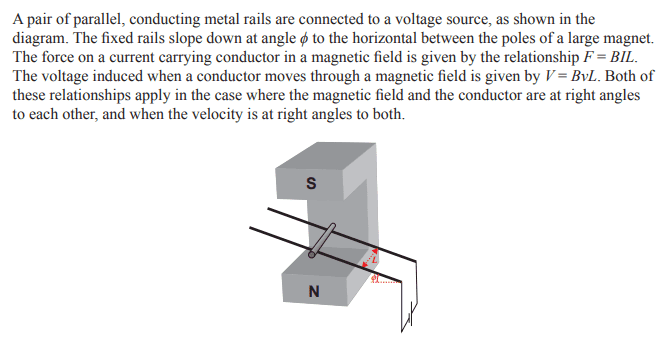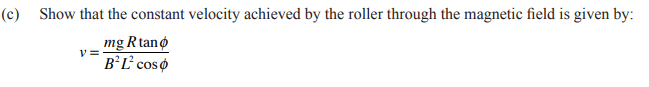My working is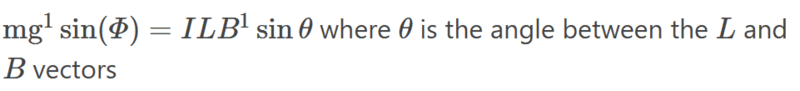However, the tricky part is to find theta. I tried to draw the situation so that I could find theta: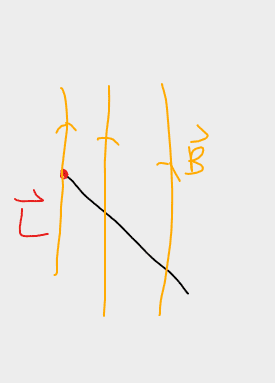It appears that theta = 90 degrees. However, this does not seem to be correct. Does anybody please know how to correctly find theta in terms of phi?

Please note I am trying to do it in using the magnetic force cross product formula while the solutions find the components of perpendicular to each other then use the magnetic force formula that is only valid when the magnetic field, current and velocity are at right angles.

Thank you!

The roller is horizontal and the field is vertical, as shown in your figure. So, the angle ##\theta## is 90 degrees, as you say.

However, your equation ##mg\sin\phi= ILB \sin \theta## is not correct. What is the direction of the magnetic force on the roller? Be sure to sketch a good free-body diagram for the roller.

The problem statement doesn't say if the roller slides or rolls along the rails. Maybe it was stated in an earlier part of the problem.

The problem statement says,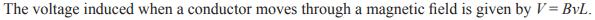This is not always true and it's not true for this problem. Do you see why? For example, what would be the induced voltage if the rod is oriented horizontally but moving vertically downward parallel to the B field?
Edit: the problem statement addresses this when it says: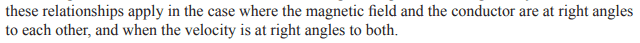But you can see that in this problem the velocity is not at right angles to B.

Is the electrical resistance of the roller given? [Edit: Nevermind, the resistance ##R## is included as part of the answer. Somehow I overlooked it.]

The figure given with the problem appears to show a battery in the rail circuit. But no mention of this is made in the problem statement.

Last edited:
•ChiralSuperfields
TSny said:
The roller is horizontal and the field is vertical, as shown in your figure. So, the angle ##\theta## is 90 degrees, as you say.

However, your equation ##mg\sin\phi= ILB \sin \theta## is not correct. What is the direction of the magnetic force on the roller? Be sure to sketch a good free-body diagram for the roller.

The problem statement doesn't say if the roller slides or rolls along the rails. Maybe it was stated in an earlier part of the problem.

The problem statement says,
View attachment 320442
This is not always true and it's not true for this problem. Do you see why? For example, what would be the induced voltage if the rod is oriented horizontally but moving vertically downward parallel to the B field?

Is the electrical resistance of the roller given?

The figure given with the problem appears to show a battery in the rail circuit. But no mention of this is made in the problem statement.

The direction of the magnetic force is up the incline at an angle phi. I got this from my free body diagram and using the right-hand rule: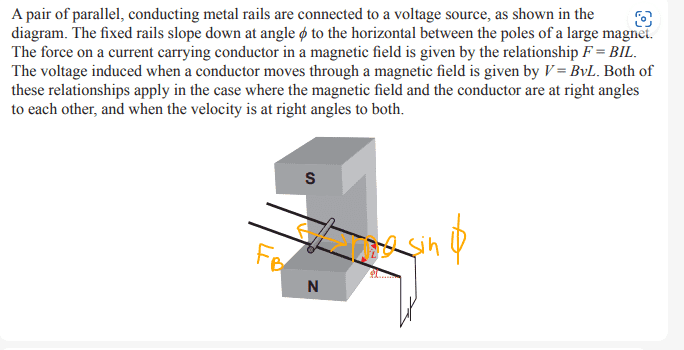Here is the earlier part of this question: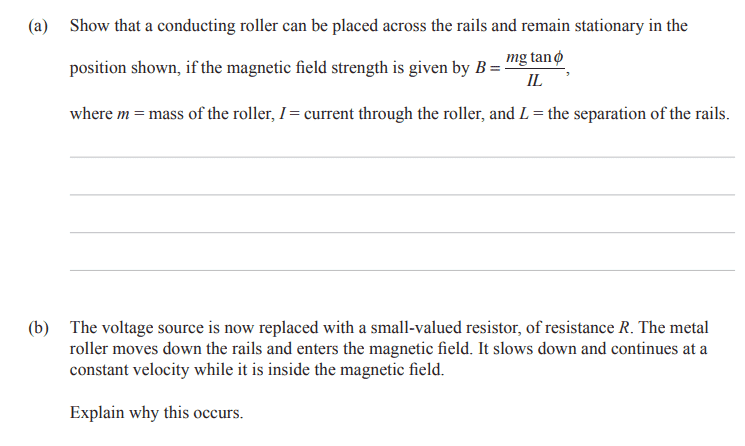Yeah, I see what mean about that V = BvL formula. I guess we will just have to find the components that are perpendicular.

Source: Q1 of https://www.nobraintoosmall.co.nz/NCEA/phySch/exams/Sch/Exams/93103-exm-2016.pdf

Many thanks!

TSny said:
The problem statement doesn't say if the roller slides or rolls along the rails.
It's not called a roller for nothing!

•ChiralSuperfields and TSny
Steve4Physics said:
It's not called a roller for nothing!But I think that the answer that's given is for the case of sliding with no friction.

Last edited:
•ChiralSuperfields
TSny said:
The figure given with the problem appears to show a battery in the rail circuit. But no mention of this is made in the problem statement.
Does it work if we replace the battery with a resistor R? There is an R in the target expression.
TSny said:But I think that the answer that's given is for the case of sliding with no friction.
Since it is constant velocity, does it matter which?

•ChiralSuperfields
haruspex said:
Does it work if we replace the battery with a resistor R? There is an R in the target expression.
I see now that according to part (c), ##R## is the resistance of a resistor that replaces the battery. So, it's OK.

haruspex said:
Since it is constant velocity, does it matter which?
I worked it for the sliding case and got the answer. I haven't worked it out for the rolling case, but it seems to me that in that case there would have to be a friction force on the roller parallel to the slope. I think this would reduce the amount of magnetic force required for constant velocity and, therefore, reduce the velocity required.

•ChiralSuperfields
TSny said:
I worked it for the sliding case and got the answer. I haven't worked it out for the rolling case, but it seems to me that in that case there would have to be a friction force on the roller parallel to the slope. I think this would reduce the amount of magnetic force required for constant velocity and, therefore, reduce the velocity required.
If it is rolling at constant velocity, why would there be a friction force?

•ChiralSuperfields and Steve4Physics
haruspex said:
If it is rolling at constant velocity, why would there be a friction force?
Ah. You're right. No torque necessary for constant velocity! Thanks.

•ChiralSuperfields and Steve4Physics
TSny said:
Ah. You're right. No torque necessary for constant velocity! Thanks.
Hi @TSny,

Could you please show me how to solve this problem using the cross-product definition of the magnetic force.

Thank you!

Callumnc1 said:
Hi @TSny,

Could you please show me how to solve this problem using the cross-product definition of the magnetic force.

Thank you!
Do you specifically want the vector version?
Have you solved it the scalar way?

•ChiralSuperfields
haruspex said:
Do you specifically want the vector version?
Have you solved it the scalar way?
Thank you @haruspex !

I am interested to know how to solve from here thanks: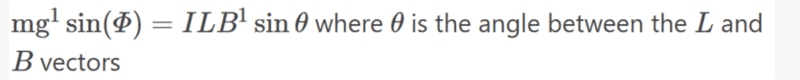Thank you so much!

Callumnc1 said:
I am interested to know how to solve from here thanks:
View attachment 320451
The equation ##mg\sin\phi = ILB\sin\theta## is not correct.
Draw a free-body diagram for the roller showing all of the forces that act on the roller.

Could you please show me how to solve this problem using the cross-product definition of the magnetic force.
The right-hand rule for the cross-product comes into play in getting the direction of the magnetic force.

#### Attachments

Last edited:
•ChiralSuperfields
TSny said:
The equation ##mg\sin\phi = ILB\sin\theta## is not correct.
Draw a free-body diagram for the roller showing all of the forces that act on the roller.

The right-hand rule for the cross-product comes into play in getting the direction of the magnetic force.
Thank you @TSny!

Here is the free body diagram,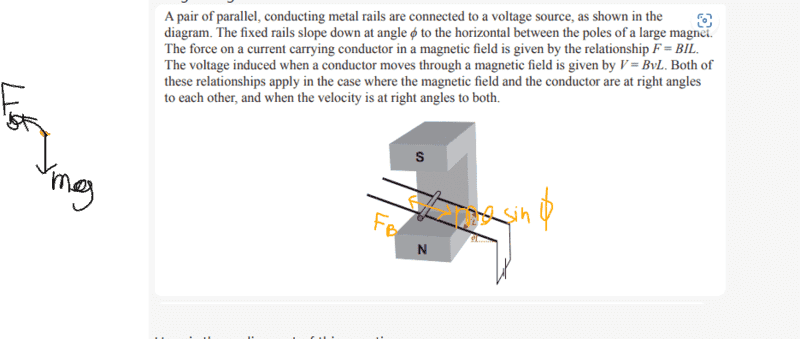Sorry it's a bit messy, so I'll put it into words. The magnetic force acts up at angle phi to the horizontal on the roller, while the weight of the roller acts vertically downwards. The normal force is perpendicular to the inclined rail (not shown in the diagram).

Many thanks!

Last edited:
Callumnc1 said:
Thank you @TSny!

Here is the free body diagram,
View attachment 320473
Sorry it's a bit messy, so I'll put it into words. The magnetic force acts up at angle phi to the horizontal on the roller, while the weight of the roller acts vertically downwards.

Many thanks!
If those are the only forces then the roller will accelerate into the rail.

•ChiralSuperfields
haruspex said:
If those are the only forces then the roller will accelerate into the rail.
Thank you @haruspex! Sorry I forgot to add the normal force which is perpendicular to the inclined rail.

Many thanks!

Callumnc1 said:
The magnetic force acts up at angle phi to the horizontal on the roller, ...

No. To get the right direction for the magnetic force on the moving roller, you first need to know which way the current flows in the roller. Here is a side view of the roller moving down the rail.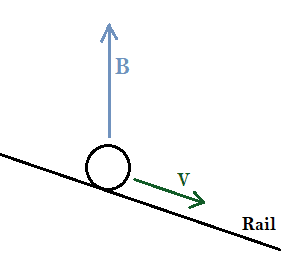Which way is the induced current flowing in the roller: into the page or out of the page? What is the reason for your answer?

•nasu and ChiralSuperfields
TSny said:
No. To get the right direction for the magnetic force on the moving roller, you first need to know which way the current flows in the roller. Here is a side view of the roller moving down the rail.
View attachment 320475

Which way is the induced current flowing in the roller: into the page or out of the page? What is the reason for your answer?
Thank for your reply @TSny! Well the battery produces an out of the page current, but I'm not sure to find the induced current in the roller given the direction of the B-field and velocity vector. Would you please be able to tell me?

Thank you again.

There is no battery for part (c) of the problem. The current in part (c) is induced by the magnetic field. One way to determine the direction of the induced current is to imagine a positive charge carrier inside the conducting roller. As the roller moves down the slope with velocity ##\vec v##, what would be the direction of the magnetic force on the charge carrier? Recall the Lorentz force law ##\vec F_B = q \vec v \times \vec B##. What is the direction of ##\vec v \times \vec B## in this picture?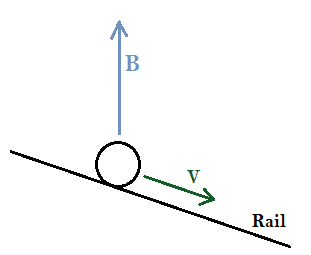Or, if you've studied Faraday's law of induction, you can get the direction of the induced current by considering Lenz's law. The roller is part of a circuit consisting of the roller, the two rails, and the resistor.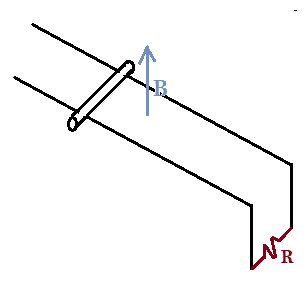The magnetic field of the magnet produces a magnetic flux through the circuit. The flux changes as the roller moves. You can use Lenz's law to determine the direction of the induced current in the circuit.

•ChiralSuperfields
TSny said:
There is no battery for part (c) of the problem. The current in part (c) is induced by the magnetic field. One way to determine the direction of the induced current is to imagine a positive charge carrier inside the conducting roller. As the roller moves down the slope with velocity ##\vec v##, what would be the direction of the magnetic force on the charge carrier? Recall the Lorentz force law ##\vec F_B = q \vec v \times \vec B##. What is the direction of ##\vec v \times \vec B## in this picture?
View attachment 320477

Or, if you've studied Faraday's law of induction, you can get the direction of the induced current by considering Lenz's law. The roller is part of a circuit consisting of the roller, the two rails, and the resistor.
View attachment 320476
The magnetic field of the magnet produces a magnetic flux through the circuit. The flux changes as the roller moves. You can use Lenz's law to determine the direction of the induced current in the circuit.
Oh, right the Lorentz force Law! Thank you @TSny !

I guess the force it out of the screen, so the current is out of the screen too.

Many thanks!

Callumnc1 said:
I guess the force it out of the screen, so the current is out of the screen too.
Yes. So, if the current in the roller is out of the page, what is the direction of the magnetic force on the current? Here, you need to consider the direction of the cross product in the force law ##\vec F = I\vec L \times \vec B##, where ##\vec L## has the direction of the current in the roller.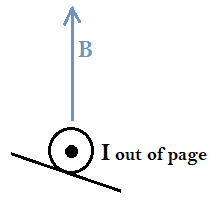TSny said:
Yes. So, if the current in the roller is out of the page, what is the direction of the magnetic force on the current? Here, you need to consider the direction of the cross product in the force law ##\vec F = I\vec L \times \vec B##, where ##\vec L## has the direction of the current in the roller.

View attachment 320478
Thanks for the reply @TSny! Up the incline. Thanks!

Last edited:
Callumnc1 said:
Up the incline.
No. You're not correctly using the right-hand rule for getting the direction of the cross product of two vectors. I'll let you work on this. I need some sleep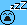•ChiralSuperfields
Callumnc1 said:
Oh, right the Lorentz force Law! … I guess the force it out of the screen
No, Lenz's law, https://en.wikipedia.org/wiki/Lenz's_law, which basically says the forces arise so as to oppose change.

In this case, we have a roller that, without the magnetic field, would accelerate downhill. So the force on the roller that results from the magnetic field will act uphill. Since that force increases with speed, it should reach a speed at which the two forces balance.

If you do all the cross products and handedness rules correctly then you should be able to confirm Lenz's law is obeyed.

•ChiralSuperfields
TSny said:
No. You're not correctly using the right-hand rule for getting the direction of the cross product of two vectors. I'll let you work on this. I need some sleepThank you for your help today @TSny and sorry for keeping you awake!

haruspex said:
No, Lenz's law, https://en.wikipedia.org/wiki/Lenz's_law, which basically says the forces arise so as to oppose change.

In this case, we have a roller that, without the magnetic field, would accelerate downhill. So the force on the roller that results from the magnetic field will act uphill. Since that force increases with speed, it should reach a speed at which the two forces balance.

If you do all the cross products and handedness rules correctly then you should be able to confirm Lenz's law is obeyed.
Ok thank for the reference about Lenz's law @haruspex !

TSny said:
Yes. So, if the current in the roller is out of the page, what is the direction of the magnetic force on the current? Here, you need to consider the direction of the cross product in the force law ##\vec F = I\vec L \times \vec B##, where ##\vec L## has the direction of the current in the roller.

View attachment 320478
I think I found my mistake when applying the right-hand rule. Here is my new free body diagram: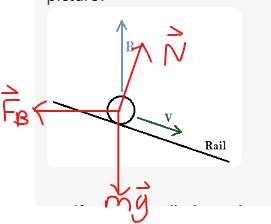From here I think I just decompose the gravitational and magnetic force into the components parallel to the incline: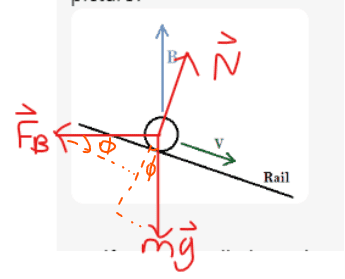Many thanks!

Callumnc1 said:
I think I found my mistake when applying the right-hand rule. Here is my new free body diagram:
View attachment 320482
From here I think I just decompose the gravitational and magnetic force into the components parallel to the incline:
View attachment 320483
Many thanks!
Looks good.

•ChiralSuperfields
Callumnc1 said:

My working is
Please learn to post math equations using LaTeX instead of posting images of your work. See the "LaTeX Guide" link below the Edit window. Thank you.

•SammyS and ChiralSuperfields
TSny said:
Looks good.
Ok thank you @TSny!

•TSny
berkeman said:
Please learn to post math equations using LaTeX instead of posting images of your work. See the "LaTeX Guide" link below the Edit window. Thank you.
Thank you @berkeman ! Unfornately I have dyslexia and dysgraphia which makes remember arbitrary strings very hard, which means that I'm not very good at coding, but I'll give it a I try.

Callumnc1 said:
but I'll give it a I try.
Thanks.I found that printing out the first few pages of that tutorial and keeping it next to my computer helps me with using LaTeX.•ChiralSuperfields
berkeman said:
I found that printing out the first few pages of that tutorial and keeping it next to my computer helps me with using LaTeX.•